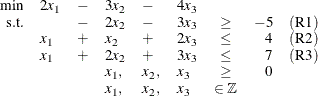# The Mixed Integer Linear Programming Solver

## Getting Started: MILP Solver

The following example illustrates how you can use the OPTMODEL procedure to solve mixed integer linear programs. For more examples, see the section Examples: MILP Solver. Suppose you want to solve the following problem:You can use the following statements to call the OPTMODEL procedure for solving mixed integer linear programs:

proc optmodel;
var x{1..3} >= 0 integer;

min f = 2*x - 3*x - 4*x;

con r1: -2*x - 3*x >= -5;
con r2: x + x + 2*x <= 4;
con r3: x + 2*x + 3*x <= 7;

solve with milp / presolver = automatic heuristics = automatic;
print x;
quit;


The PRESOLVER= and HEURISTICS= options specify the levels for presolving and applying heuristics, respectively. In this example, each option is set to its default value, AUTOMATIC, meaning that the solver automatically determines the appropriate levels for presolve and heuristics.

The optimal value of x is shown in Figure 7.1.

Figure 7.1: Solution Output

The OPTMODEL Procedure

 x
1 0
2 1
3 1

The solution summary stored in the macro variable _OROPTMODEL_ can be viewed by issuing the following statement:

%put &_OROPTMODEL_;


This statement produces the output shown in Figure 7.2.

Figure 7.2: Macro Output

 STATUS=OK ALGORITHM=BAC SOLUTION_STATUS=OPTIMAL OBJECTIVE=-7 RELATIVE_GAP=0 ABSOLUTE_GAP=0 PRIMAL_INFEASIBILITY=0 BOUND_INFEASIBILITY=0 INTEGER_INFEASIBILITY=0 BEST_BOUND=-7 NODES=1 ITERATIONS=5 PRESOLVE_TIME=0.00 SOLUTION_TIME=0.05